# Simple Linear Regression

We live in a world that is filled with patterns – patterns all around us just waiting to be discovered. Some of these patterns are not as easily discovered because of the existence of outside noise.

Consider for example an experiment where a set of people were each given the task of drinking a number of beers and having their blood alcohol level taken afterwards. Some noise factors in this could include the height and weight of the individual, the types of drinks, the amount of food eaten, and the time between drinks. Even with this noise, though, we can still see a correlation between the number of drinks and their blood alcohol level. Consider the following graph showing people’s blood-alcohol level after a given number of drinks. The x-axis represents the number of drinks and the y-axis is the corresponding blood alcohol level.x 5 2 9 8 3 7 3 5 3 5 4 6 5 7 1 4 y 0.1 0.03 0.19 0.12 0.04 0.095 0.07 0.06 0.02 0.05 0.07 0.1 0.085 0.09 0.01 0.05

We can definitely see a correlation, and although the data doesn’t quite fit on a straight line. It leads us to ask further questions like can we use this data to build a model that estimates a person’s blood-alcohol level and how strong is this model?

One of the tools we can use to model this problem is linear regression. A linear regression takes a two-dimensional data set, with the assumption that one column (generally represented by the x variable) is independent and the second column (generally represented by the y variable) being dependent on the first column. The assumption is that the relationship between the two columns is linear and can be represented by the linear equation

y =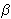0 +1x + e.

The right hand side of the above equation has three terms. The first two (0 and1) are the parameters of the linear equation (the y-intercept and slope respectively), while the third term of the right hand side of the above equation represents the error term. The error term represents the difference between this linear equation and the y values in the data provided. We are seeking a line that minimizes the error term. That is, we are seeking to minimize

D =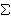i = 1 to n [yi – (0 +1xi)]2

There are several ways one could approach this problem. In fact, there are several lines that one could use to build a linear model. The first line that one may use to model these points is the one generated by only mean of the y values of the points, called the horizontal line regression.

For the data set above, the mean of the y values can be calculated as= 0.0738, so we could build a linear model based on this mean that would be y = 0.738. This horizontal line regression model is a horizontal line that predicts the same score (the mean), regardless of the x value. This lack of adjustments means it is generally a poor fit for most models. But as we will see later, this horizontal line regression model does serve a purpose in determining how well the model we develop performs.

A second attempt at solving this problem would be to generate the least squares line. This is the line that minimizes the D value listed above. We can see that D is a multi-variable polynomial, and we can find the minimum of such a polynomial using calculus, partial derivatives and Gaussian elimination (I will omit the work here because it deters us from the main point of this blog post, however Steven J. Miller has a good write-up of this).

The calculus leads us to the following equations:

SXY =i = 1 to n(xy) –
 (i = 1 to nx)(i = 1 to ny) n
SXX =i = 1 to n(x2) –
 (i = 1 to nx)2 n1 =
 SXY SXX0 =–1To calculate the least squares line for this example, we first need to calculate a few values:i = 1 to n(xy) = 6.98i = 1 to n(x2) = 443i = 1 to nx = 77i = 1 to ny = 1.18
Sxx = 72.44
Sxy = 1.30

This lets us evaluate that1 = 0.018
and0 = -0.0127

So the resulting linear equation for this data is= -0.0127 + 0.018*x

Below is a graph of the two attempts at building a linear model for this data.In the above image, the green line represents the horizontal line regression model and the blue line represents the least-squares line. As stated above, the horizontal line regression model is a horizontal line that does not adjust as the data changes. The least-squares line adjusts both the slope and y-intercept of this line according to the data provided to better fit the data provided. The question becomes how well does the least-squares line fit the data.

The Sum of Squares Error (SSE) sums the deviation at each point of our data from the least-squares line.

SSE =i = 1 to n(yii)2

A second metric that we are interested in is how well the horizontal line regression linear model estimates our data. This is called the Total Sum of Squares (SST).

SST =i = 1 to n(yi)2

The horizontal line regression model ignores the independent variable x from our data set and thus any line that takes this independent variable into account will be an improvement on the horizontal line regression model. Because of this, the SST sum is a worse case scenario of how poorly our model can perform.

Knowing now that SST is always greater than SSE, the regression sum of squares (SSR) is the difference between the total sum of squares and the sum of squares error.

SSR = SST – SSE

This tells us how much of the total sum of squares is accounted for by the model.

Finally, the coefficient of determination (r2) is defined by

r2 = SSR / SST

This tells SSR as a percentage of SST, or the amount of the variation in the data that is explained by the model.

So, check out my script on simple linear regression and let me know what you think.

# Covariance of VectorsMost of the things we think about have many different ways we could describe them. Take for example a movie. I could describe a movie by its genre, its length, the number of people in the movie, the number of award winners, the length of the explosions, the number of fight scenes, the number of scenes, the rating it was given by a certain critic, etc. The list goes on and on. How much do these things influence one another? How likely is a person to enjoy a movie? Is that related to the number of award winners in the movie? Answering this type of a question can often help understand things like what might influence a critics rating or more importantly which movies are worth my \$15 ticket price.

Movies are just one example of this. Other areas like sports, traffic congestion, or food and a number of others can be analyzed in a similar manner. With data becoming available at unprecedented rates and areas like cloud computing and data science becoming key buzzwords in industry, the ability to understand these relationships is becoming more and more important.

As a mathematician, I enjoy being able to say with certainty that some known truth is the cause of some other known truth, but it not always easy (or even possible) to prove the existence of such a relationship. We are left instead with looking at trends in data to see how similar things are to one another over a data set. Measuring the covariance of two or more vectors is one such way of seeking this similarity.

Before delving into covariance though, I want to give a refresher on some other data measurements that are important to understanding covariance.
– Sum of a vector:
If we are given a vector of finite length we can determine its sum by adding together all the elements in this vector. For example, consider the vector v = (1, 4, -3, 22). Then sum(v) = 1 + 4 + -3 + 22 = 24.

– Length of a vector:
If we are given a vector of finite length, we call the number of elements in the vector the length of the vector. So for the example above with the vector v = (1, 4, -3, 22), there are four elements in this vector, so length(v) = 4.

– Mean of a vector:
The mean of a finite vector is determined by calculating the sum and dividing this sum by the length of the vector. So, working with the vector above, we already calculated the sum as 24 and the length as 4, which we can use to calculate the mean as the sum divided by the length, or 24 / 4 = 6.

– Variance of a vector:
Once we know the mean of a vector, we are also interested in determining how the values of this vector are distributed across its domain. The variance measures this by calculating the average deviation from the mean. Here we calculate the deviation from the mean for the ith element of the vector v as (vi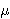)2. We can get the average deviation from the mean then by computing the average of these values.

So if the vector v has n elements, then the variance of v can be calculated as Var(v) = (1/n)i = 1 to n((vi)2).

Once again dealing with the vector above with v = (1, 4, -3, 22), where the mean is 6, we can calculate the variance as follows:

 vi vi –(vi –)2 1 -5 25 4 -2 4 -3 -9 81 22 16 256

To calculate the mean of this new vector (25, 4, 81, 324), we first calculate the sum as 25 + 4 + 81 + 256 = 366. Since the length of the new vector is the same as the length of the original vector, 4, we can calculate the mean as 366 / 4 = 91.5

The covariance of two vectors is very similar to this last concept. Instead of being interested in how one vector is distributed across its domain as is the case with variance, covariance is interested in how two vectors X and Y of the same size are distributed across their respective means. What we are able to determine with covariance is things like how likely a change in one vector is to imply change in the other vector. Having a positive covariance means that as the value of X increases, so does the value of Y. Negative covariance says that as the value of X increases, the value of Y decreases. Having zero covariance means that a change in the vector X is not likely to affect the vector Y.

With that being said, here is the procedure for calculating the covariance of two vectors. Notice that it is very similar to the procedure for calculating the variance of two vectors described above. As I describe the procedure, I will also demonstrate each step with a second vector, x = (11, 9, 24, 4)

1. Calculate the means of the vectors.
As we’ve seen above, the mean of v is 6.
We can similarly calculate the mean of x as 11 + 9 + 24 + 4 = 48 / 4 = 12

2. Subtract the means of the vectors from each element of the vector (xiX) and (YiY).

We did this for v above when we calculated the variance. Below are the values for v and for x as well.

vi – meanv

-5

-2

-9

16

 i vi xi xi – meanx 1 1 11 -1 2 4 9 -3 3 -3 24 12 4 22 4 -8

3. For each element i, multiply the terms (xiX) and (YiY).

This gives us the following vector in our example:
(-5)(-1), (-2)(-3), (-9)(12), (16)(-8) = (5, 6, -108, -128).

4. Sum the elements obtained in step 3 and divide this number by the total number of elements in the vector X (which is equal to the number of elements in the vector Y).

When we sum the vector from step 3, we wind up with 5 + 6 + -108 + -128 = -225
And the result of dividing -225 by 4 gives us -225/4 = – 56.25.

This final number, which for our example is -56.25, is the covariance.

Some important things to note are

• If the covariance of two vectors is positive, then as one variable increases, so does the other.
• If the covariance of two vectors is negative, then as one variable increases, the other decreases.
• If the covariance of two vectors is 0, then one variable increasing (decreasing) does not impact the other.
• The larger the absolute value of the covariance, the more often the two vectors take large steps at the same time.
• A low covariance does not necessarly mean that the two variables are independent. I’ll give a quick example to illustrate that.
Consider the vectors x and y given by x = (-3, -2, -1, 0, 1, 2, 3) and y = (9, 4, 1, 0, 1, 4, 9).
The mean of x is 0, while the mean of y is 7.
The mean adjusted values are (-3, -2, -1, 0, 1, 2, 3) and (2, -3, -6, -7, -6, -3, 2).
The product of these mean adjusted values is (-6, 6, 6, 0, -6, -6, 6).
If we sum this last vector, we get 0, which after dividing by 7 still gives a value of 0.
So the covariance of these two vectors is 0.

We can easily see that for each value xi in x, the corresponding yi is equal to xi2

I have written a script to help understand the calculation of two vectors.

# The Gram-Schmidt Process and Orthogonal Vectors

Suppose I gave you some red fingerpaint and asked you to make all the colors you could from this paint. You’d probably come up with a diverse collection of pinks, reds and burgendys – going through the range of reds – but you would be unable to produce a color that does not depend solely on red, like purple.If I were to ask you to produce purple, your reply may be something like “well, give me blue and I’ll be able to color in purple”. When we think in terms of colors, it is easy to understand the concept of the span of a set of colors. In this context, span refers to the set of colors we can create from our original colors.

Now, lets think in terms of numbers (actually vectors) instead of colors. If I gave you a similar task as above, but instead of the color red, I gave you the vector (1, 0, 0) and told you to see what other numbers you could get from this (by scalar multiplication and the addition of any two vectors already produced) then you would probably come back to me and show me how you could use the vector (1, 0, 0) to produce the entire real number line in that first dimension, but leaving the other coordinates at 0.

If (similar to the color example) I asked you to use the vector (1, 0, 0) to produce the vector (1, 1, 0), then you may reply with a similar statement as above: “you give me the vector (0, 1, 0) and I’ll produce (1, 1, 0)”. That’s because the vectors (1, 0, 0) and (1, 1, 0) are linearly independent. This is a mathematical way of saying what we’ve already stated, that you cannot get one vector as a scalar multiple of the other vector for any real number scalar.

If two vectors are linearly independent then neither one belongs to the span of the other. Just as you cannot create blue from red, you cannot create red from blue. So this means that if I were to give you the colors red and blue, then the set of colors that you can create has increased from what you could create from either only red or only blue. Similarly, the vector sets {(1, 0, 0), (0, 1, 0)} spans more vectors than just {(1, 0, 0)} or {(0, 1, 0)}.

Consider then the following two sets of vectors: {(1, 0, 0), (0, 1, 0)} and {(1, 0, 0), (1, 1, 0)}. Notice that (1, 1, 0) [belongs to] span({(1, 0, 0), (0, 1, 0)}) because (1, 1, 0) = (1, 0, 0) + (0, 1, 0). Likewise (0, 1, 0) [belongs to] span({(1, 0, 0), (1, 1, 0) because (0, 1, 0) = (1, 1, 0) – (1, 0, 0). So we can see that these two sets span the same sets of vectors. But which is a better set to use?

Lets to back to colors and think of the set {red, purple}. Here we can think of purple as a simple combination of red and blue (i.e. purple = red + blue). What happens if we mix red and purple? If we think of it in terms of an axis, the fact that purple contains red in it means that as we walk along the purple axis, we are walking along the red axis as well. If we considered the set {red, blue}, we see that this is not happening. As we change the amount of red in our color, the amount of blue is unaffected. Likewise, if we change the amount of blue, the amount of red is not affected. If we were to draw these axes, we could see that this happens because the colors red and blue are perpendicular (or orthogonal) to one another, while red and purple are not.Replacing these colors with vectors again, we get the same thing. We have the option of using the vector set {(1, 0, 0), (1, 1, 0)} or {(1, 0, 0), (0, 1, 0)} as our axis and it is better to use the second set because the two vectors are not just independent of one another, but also are at a 90[degree] angle, or are orthogonal to one another.

When given a set of vectors (or a set of colors), an important problem is to determine the span of those vectors and to be able to produce an orthogonal set of vectors that spans that same space. The Gram-Schmidt process provides a procedure for producing these orthogonal vectors.

The way the procedure works is to build an orthogonal set of vectors from the original set by computing the projection of the current vector being worked on in terms of the previous vectors in the orthogonal set. This projection procedure is defined as proju(v) = (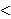u, v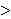/u, u)u. The formula for the ith vector of the Gram-Schmidt process is

ui = vi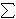j = 1 to i-1 projuj(vi).

Here is a script I’ve written to help with this process.

# Gaussian Elimination

I have just written a script which executes the Gaussian Elimination Algorithm.

When we have a collection of lines we wish to know if they all intersect at some point. Many times we are interested in determining what that point is. In order to calculate this information, we first need an understanding of the lines themselves. The way the Gaussian Elimination Algorithm works is that the collection of lines are input using a notation of Ax = b, where the matrix A is called the coefficient matrix, as the nth row of it corresponds to the coefficients for the nth line being considered. The vector b represents the right hand side vector (in two dimensions, we would call these constants the y-intercepts of the lines. In higher dimensions they hold a similar property). The vector x represents the point where the lines intersect. It is this quantity which Gaussian Elimination seeks to determine.

The basic procedure of Gaussian Elimination is to use \”elementary row operations\” on the matrix (A|b), which is called the augmented matrix, to transform A into upper triangular form. Once this is done, a procedure called back-substitution can find the solution (x) to this problem.

The elementary row operations that we are allowed to perform are:

• Interchange two rows.
• Multiply a row by a nonzero number.
• Add a row to another one multiplied by a number.
• For the last property listed above, we will determine this number by dividing the coefficient of the term we which to eliminate by the negative of the coefficient of the element on the main diagonal of the same column of the matrix. This will have the property of cancelling out, or producing a desired zero in the resulting row.

If this algorithm produces an upper triangular matrix from which we can solve for x using back-substitution. This procedure of back-substitution is simply solving for the vector x from the bottom of the matrix to the top. If the algorithm does not produce an upper triangular matrix (because somewhere along the line, we are unable to obtain a ratio because we have zero’s on the diagonal and all zeros below the diagonal), then we say the matrix is singular. This means that there is no unique point where the lines all intersect.

To learn more and see more examples, check out My Script on Gaussian Elimination.